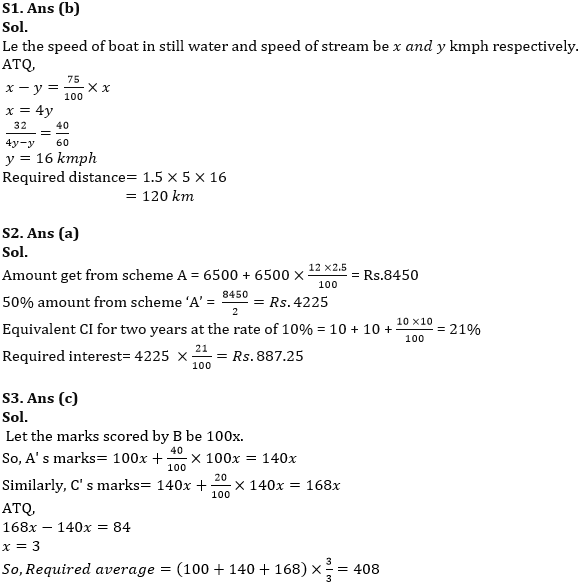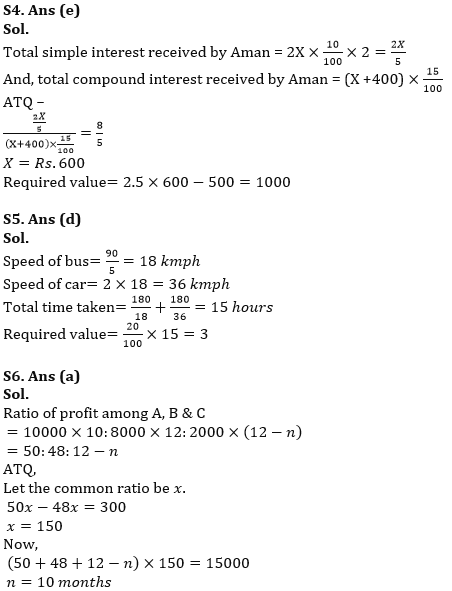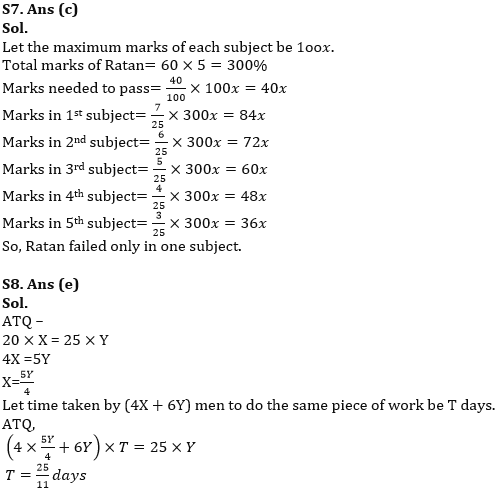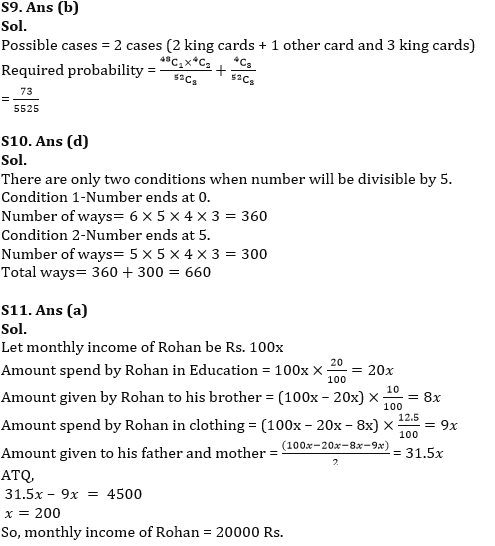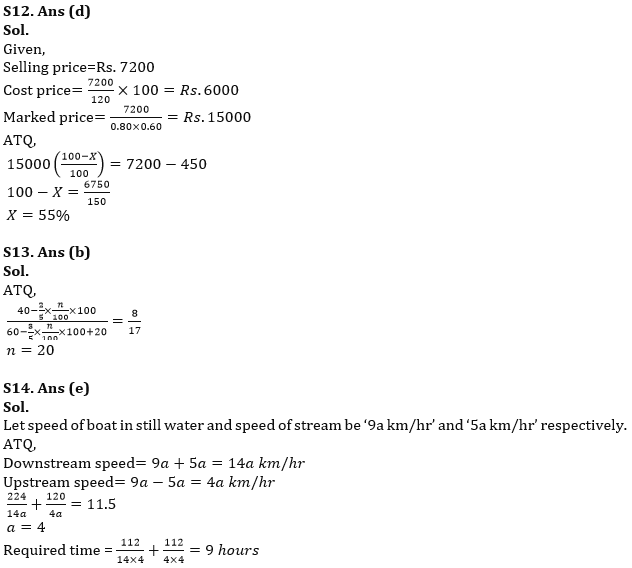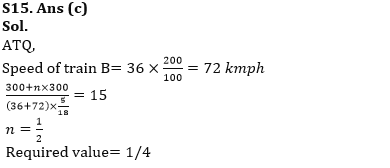Latest Banking jobs   »   SEBI Grade A syllabus 2022   »

# Quantitative Aptitude Quiz For RBI Grade B/ ECGC PO/ SIDBI Grade A Prelims 2022- 7th April

Q1. The upstream speed of a boat is 25% less than speed of boat in still water and boat cover 32 km distance in upstream in 40 minutes. Find the distance covered by boat in 1.5 hour going downstream? (in km)
(a)100
(b)120
(c)140
(d)180
(e)80

Q2. A sum of Rs.6500 invested in scheme ‘A’ at the rate of 12% p.a. for 2.5 years at simple interest p.a. If 50% amount received from scheme ‘A’ is invested in scheme ‘B’ offering compound interest at the rate of 10% p.a. for 2 years, then find the compound interest received at the end of 2 years? (in Rs.)
(a)887.25
(b)900.50
(c)872.75
(d)908.75
(e)935.50

Q3. The marks scored by A is 40% more than B’s marks. C got 20% more marks than A. If difference between marks scored by A and C is 84, then find the average marks scored by all three.
(a)364
(b)386
(c)408
(d)432
(e)470

Q4. Aman invested Rs.2X at the rate of 10% p.a. at simple interest for two years and Rs. (X +400) at the rate of 15% at compound interest for one year. If ratio of total simple interest to total compound interest received by Aman is 8: 5, then what is the value of 2.5X-500?
(a)1525
(b)800
(c)1225
(d)775
(e)1000

Q5. If a bus travels 90 km in 5 hours and ratio of speed of bus to car is 1:2, then the total time taken by both bus and car to travel 180 km individually is ‘n’ hours. Find the value of 20% of n?
(a)12
(b)6
(c)9
(d)3
(e)15

Q6. A and B started a business with the initial capital of Rs.10000 and Rs.8000 respectively. After ‘n’ months, C joined them with the initial capital of Rs.2000. A invested for only 10 months. If at the end of a year total profit of business is Rs.15000 and profit share of B is Rs.300 less than that of A. Find the value of n?
(a)10
(b)9
(c)4
(d)5
(e)6

Q7. Ratan gets an aggregate of 60% marks in five subjects in ratio 7: 6: 5: 4: 3. Maximum marks of each subject is same and 40% of maximum marks is needed to pass each exam. Find in how many subjects did he fail the examination?
(a)5
(b)4
(c)1
(d)2
(e)3

Q8. X men can do a piece of work in 20 days while Y men can do the same piece of work in 25 days. In how many days (4X + 6Y) men will do same piece of work? (in days)
(a)17/7
(b)30/19
(c)18/11
(d)26/17
(e)25/11

Q9. Find the probability of getting at least two king cards if three cards are drawn randomly from a pack of 52 cards?
(a)79/5321
(b)73/5525
(c)83/5321
(d)91/5525
(e)97/5321

Q10. How many different 5-digit numbers can be formed using digits 0, 1, 2, 3, 4, 5, 6 such that no digit repeats and each number so formed is divisible by 5?
(a)620
(b)740
(c)720
(d)660
(e)600

Q11. Rohan spends 20% of his income in Education, 10% of remaining given to his brother, 12.5% of remaining spend in clothing. If Rohan divided remaining income between his father and mother equally and difference between amount spend by Rohan in clothing and amount given to his father is Rs. 4500, then find monthly income of Rohan (in Rs.)?
(a)20000
(b)12000
(c)16000
(d)24000
(e)28000

Q12. A shopkeeper allows two consecutive discounts, 20% and 40% on marked price of an article and still he makes a profit of 20% by selling it. He would make Rs.450 less profit if he allows a single discount of X% on same article. Find value of X if initial selling price of article is Rs.7200?
(a)70
(b)45
(c)75
(d)55
(e)65

Q13. There is a 100 liters mixture of alcohol and water in the ratio of 2:3 . If n% of mixture is taken out and 20 liters of water is added, then ratio of water to alcohol changes to 17: 8, then find the value of n?
(a)120
(b)20
(c)25
(d)50
(e)75

Q14. The ratio of speed of boat in still water to speed of stream is 9: 5 and total time taken by boat to cover 224 km in downstream and 120 km in upstream in 11.5 hours. Find the total time taken by boat to cover 112 km each in downstream and in upstream? (in hours)
(a)18
(b)12
(c)16
(d)14
(e)9

Q15. Train ‘A’ having length 300 meters running at a speed of 36 kmph crosses another train ‘B’ which length ‘n’ times of A’s length running in opposite direction at a speed 200% of train A’s speed in 15 seconds. Find the value of n²?
(a)9/16
(b)4/9
(c)1/4
(d)1/2
(e)1/9

Solutions